Test: Plane Waves

# Test: Plane Waves

Test Description

## 10 Questions MCQ Test Electromagnetics | Test: Plane Waves

Test: Plane Waves for Electronics and Communication Engineering (ECE) 2023 is part of Electromagnetics preparation. The Test: Plane Waves questions and answers have been prepared according to the Electronics and Communication Engineering (ECE) exam syllabus.The Test: Plane Waves MCQs are made for Electronics and Communication Engineering (ECE) 2023 Exam. Find important definitions, questions, notes, meanings, examples, exercises, MCQs and online tests for Test: Plane Waves below.
Solutions of Test: Plane Waves questions in English are available as part of our Electromagnetics for Electronics and Communication Engineering (ECE) & Test: Plane Waves solutions in Hindi for Electromagnetics course. Download more important topics, notes, lectures and mock test series for Electronics and Communication Engineering (ECE) Exam by signing up for free. Attempt Test: Plane Waves | 10 questions in 30 minutes | Mock test for Electronics and Communication Engineering (ECE) preparation | Free important questions MCQ to study Electromagnetics for Electronics and Communication Engineering (ECE) Exam | Download free PDF with solutions
 1 Crore+ students have signed up on EduRev. Have you?
Test: Plane Waves - Question 1

### Which of the following has the lowest frequency?

Detailed Solution for Test: Plane Waves - Question 1

1. Under the electromagnetic spectrum, visible light has the lowest frequency among the given option.

2. The spectrum ranges from radio waves having low frequency till gamma rays, having the highest one.

Test: Plane Waves - Question 2

### For a plane wave propagating in an unbounded medium (say, free space), the minimum angle between electric field and magnetic field vectors is

Detailed Solution for Test: Plane Waves - Question 2

Concept:

Loss tangent is given by

tan⁡θ = σ/ω

And angle between E and H is:
θn  = θ/2

For a plane wave propagating in an unbounded medium, the angle between the electric field and magnetic field is always 90°

Test: Plane Waves - Question 3

### A uniform plane wave propagating in a medium has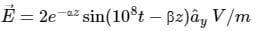if the medium is characterized by ϵr = 1, μr = 20 and σ = 3 S/m. The value of α and β are respectively.

Detailed Solution for Test: Plane Waves - Question 3

Concept:

The waves are used for the transportation of energy or information.

Characteristics of a plane wave in good conductors.

In a good conductor σ >> ωϵ

σ ≈ ∞, ϵ = ϵ0, μ = μ0μr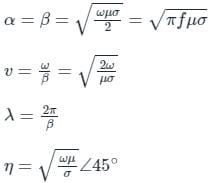Calculation:

Given ϵr = 1, μr = 20, σ = 3 S/m and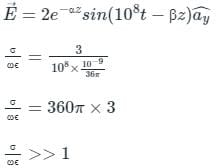Hence the given medium is a good conductor.

In a good conductor the values of α and β are: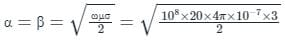α = β = 61.39

Test: Plane Waves - Question 4

When an electromagnetic wave is incident on an object having surface roughness comparable to the wavelength, then:

Detailed Solution for Test: Plane Waves - Question 4

Concept:

When more than one incident light ray or any EM wave, reflects off a surface, the laws of reflection still holds, but the surface affects the way we see the reflected rays.

Specular Reflection:

It happens when a beam of light ‘or’ EM waves in general, is reflected “perfectly” (in parallel to each other) from a shiny, polished surface. Ex- mirrors, stainless steel, glass, etc.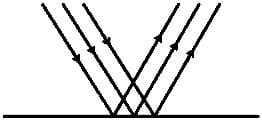Diffuse Reflection:

It happens when a Beam of light is Reflected “irregularly” (in different directions) from an irregular surface. Ex- sheet fo paper, tabletop etc.

This is explained with the help of the diagram shown:-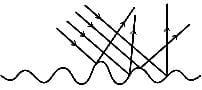Test: Plane Waves - Question 5

The expression for velocity of a wave in the conductor is

Detailed Solution for Test: Plane Waves - Question 5

The velocity is the ratio of the frequency to the phase constant.
In conductors, the phase constant is given by √(ωμσ/2).
On substituting for β,ω in v, we get v = √(2ω/μσ) units.

Test: Plane Waves - Question 6

The velocity of a wave travelling in the air medium without transmission lines or waveguides(wireless) is

Detailed Solution for Test: Plane Waves - Question 6

In free space or air medium, the velocity of the wave propagating will be same as that of the light.
Thus the velocity is the speed of light,
V = c. It is given by 3 x 108m/s.

Test: Plane Waves - Question 7

Which parameter is unity in air medium?

Detailed Solution for Test: Plane Waves - Question 7

In free space or air medium, the relative permeability is also unity, like relative permittivity.
The absolute permeability is given by 4π x 10-7 units.

Test: Plane Waves - Question 8

The total permeability in a conductor is

Detailed Solution for Test: Plane Waves - Question 8

The total permeability is the product of the absolute and the relative permeability.
For metals or conductors, the relative permittivity is not unity.
Thus the permittivity is the product of absolute and relative permeability.

Test: Plane Waves - Question 9

The intrinsic impedance of free space is

Detailed Solution for Test: Plane Waves - Question 9

The intrinsic impedance is the square root of ratio of the permeability to the permittivity. In free space, the permeability and the permittivity is same as the absolute permeability and permittivity respectively.
This is due to unity permeability and permittivity in free space.
Thus η = √(μ/ε), where absolute permeability is given by 4π x 10-7 and absolute permittivity is given by 8.854 x 10-12.
The intrinsic impedance will be 377 ohms.

Test: Plane Waves - Question 10

The phase shift in the electric and magnetic fields in an EM wave is given by which parameter?

Detailed Solution for Test: Plane Waves - Question 10

The intrinsic impedance in a conductor is given by η = √(ωμ/2σ) x (1 + j).
The phase shift is represented by the 1 + j term.
In polar form it indicates 45 degree phase shift.

## Electromagnetics

11 videos|64 docs|88 tests
Information about Test: Plane Waves Page
In this test you can find the Exam questions for Test: Plane Waves solved & explained in the simplest way possible. Besides giving Questions and answers for Test: Plane Waves, EduRev gives you an ample number of Online tests for practice

## Electromagnetics

11 videos|64 docs|88 tests(Scan QR code)